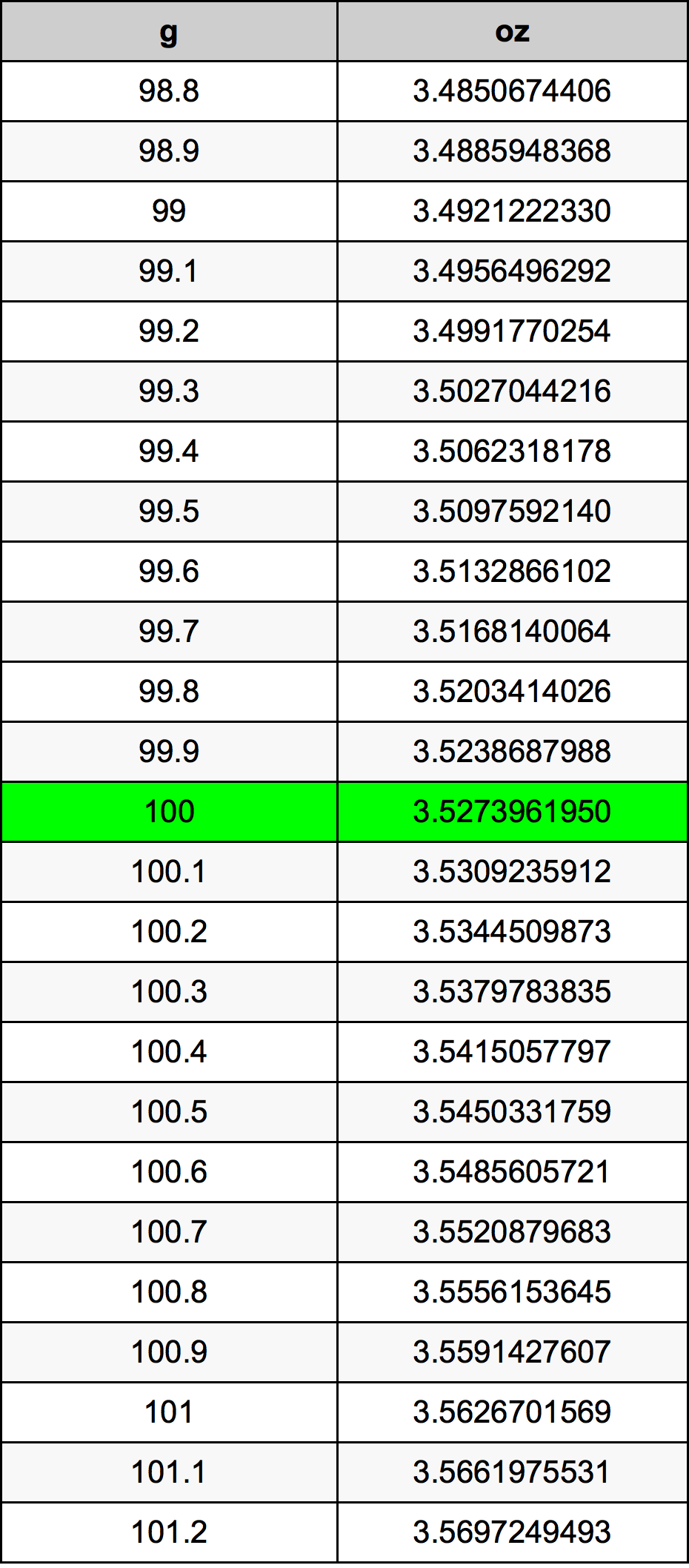Grams To Ounces

# 100 g to oz100 Grams to Ounces

g
=
oz

## How to convert 100 grams to ounces?

 100 g * 0.0352739619 oz = 3.527396195 oz 1 g
A common question is How many gram in 100 ounce? And the answer is 2834.9523125 g in 100 oz. Likewise the question how many ounce in 100 gram has the answer of 3.527396195 oz in 100 g.

## How much are 100 grams in ounces?

100 grams equal 3.527396195 ounces (100g = 3.527396195oz). Converting 100 g to oz is easy. Simply use our calculator above, or apply the formula to change the length 100 g to oz.

## Convert 100 g to common mass

UnitMass
Microgram100000000.0 µg
Milligram100000.0 mg
Gram100.0 g
Ounce3.527396195 oz
Pound0.2204622622 lbs
Kilogram0.1 kg
Stone0.0157473044 st
US ton0.0001102311 ton
Tonne0.0001 t
Imperial ton9.84207e-05 Long tons

## What is 100 grams in oz?

To convert 100 g to oz multiply the mass in grams by 0.0352739619. The 100 g in oz formula is [oz] = 100 * 0.0352739619. Thus, for 100 grams in ounce we get 3.527396195 oz.

## 100 Gram Conversion Table## Alternative spelling

100 Gram to Ounce, 100 Gram in Ounce, 100 Gram to oz, 100 Gram in oz, 100 Grams to Ounces, 100 Grams in Ounces, 100 Grams to Ounce, 100 Grams in Ounce, 100 g to oz, 100 g in oz, 100 Gram to Ounces, 100 Gram in Ounces, 100 g to Ounce, 100 g in Ounce